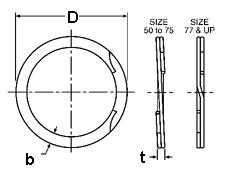### RRN-1325

Display Units: inches |  metric
Request Quick Quote for this part.
 Ring Specs (D) Free Diameter: 13.943 +0.140 / -0.000 in. (t) Thickness: 0.187 +0.005 / -0.005 in. (b) Radial Wall: 0.662 +0.005 / -0.010 in. Groove Specs: (B) Application Diameter: 13.250 in. (G) Groove Diameter: 13.846 +0.012 / -0.012 in. (W) Groove Width: 0.209 +0.008 / -0.000 in. Groove Depth: 0.298 in. Other Specs Approximate Weight per 1000: (m) Material Thickness: 0.092 in. (N) Ring Number of Turns: 2.000 (Pg) Theoretical Thrust Load Capacity - Groove Yield:Notes: Yield Strength of Groove Material (Ys): 45,000 psi. Calculated using a safety factor (K) of 2Equation:Pg = [ B * d * Ys * pi ] / K    = [ (13.250 in.) * (0.298 in.) * (45,000 psi) * 3.14 ] / 2 278,961 lbs. (Pr) Theoretical Thrust Load Capacity - Ring Shear:Notes: Ring Material: Carbon Spring Steel (SAE 1070-1090). Shear Strength of Ring Material (Ss): 120,000 psi. Calculated using a safety factor (K) of 3Equation:Pr = [ B * t * Ss * pi ] / K    = [ (13.250 in.) * (0.187 in.) * (120,000 psi) * 3.14 ] / 3 311,205 lbs. Industry Equivalent Part Number(s):RRN-1325, KG-1325, WHM-1325